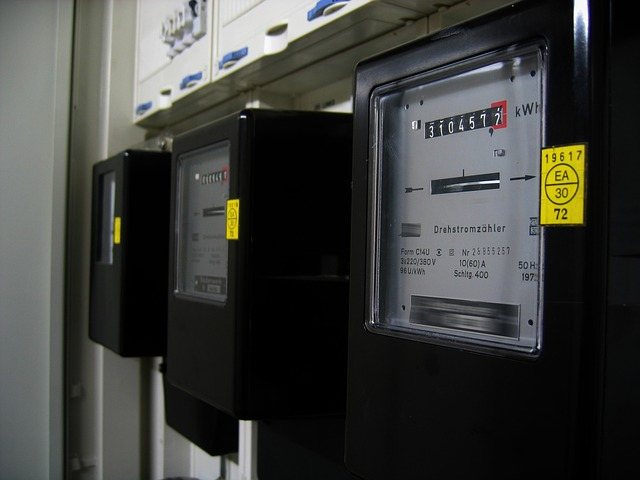Home Electrical Effect of Low / High Voltage on Electricity Bill

# Effect of Low / High Voltage on Electricity Bill

3715Effect of low/ high voltage on electricity bill- have you ever thought about this?  Does high voltage causes more consumption of electricity? Let’s learn.

Voltage and current are crying needs for a device operation. Voltage is very crucial to deviate electrons from the conductor connected to your device. Again, the flow of electrons is the current.. Current-voltage pairing initiates quite a bit of love for each other. One cannot live without the other.

If you give too much current and zero voltage then no device will work. Again, if you give too much voltage and zero amount of current, no device will work. You must provide both to operate an electrical device.

Furthermore, with this union of the couple Current-Voltage gives birth to their son power. Again, this power is the product of current and voltage.

## How is the electricity bill calculated?

When calculating the electricity bill, the son of the voltage-current pair (power) is given priority. That means based on the amount of utilization of the power at a given time. Now, with this kilowatt-hour, the calculation of our entire electricity bill takes place. Have you ever thought about the effect of low/ high voltage on electricity bill.

## Kilowatt-hours

A simple unit of electrical energy measurement. In electric lamps, you can see the writings 25, 40, 60, 100 watts, etc. to measure the amount of electrical energy converted into other energy at a particular time.

Moreover, this Watt is the child of voltage and current i.e. their product. A watt is a small unit, so a kilowatt unit is more in use for large machinery engines, etc. One kilowatt-hour is the amount of energy consumed by a device running for one hour. Billing is also calculated in kilowatt-hours.

If an 80-watt electric fan runs for 10 hours, the cost will be 80 watts x 10 hours = 800 watts-hours or 0.8 kW-hours. Again, if a light with 60 watts is lit for 24 hours, the cost will be 60 watts x 24 hours = 1440 watts-hours or 1.44 kilowatt-hours. So if the price per unit is 5 USD then it will cost 0.8 x 5 = 4 USD to run the fan for 10 hours. Furthermore, to run the light for 24 hours it will cost 1.44 x 5 = 7.20 USD.

## Effect on Electricity Bill due to decrease in Voltage

Each device has a certain amount of voltage rating. Normally the device is capable of full operation only when the rated voltage is applied. However, if the voltage decreases, there will be no effect on the electricity bill. Because the electricity bill is not calculated on the volt. It depends on how much electrical energy the device has converted into other energy at a given time.

## Calculations through real-life examples

Suppose, tomorrow is your semester final exam. So, you leaned your head in front of the table lamp of 26 watts and 220-volt rating. There you studied straight for 3 hours with great care. Now, we will calculate the electricity bill for these three hours. Since the table lamp is 26 watts and the voltage rating is 220 volts, it will take current = 26/220 = 0.12 amperes. Then the output power = 0.12 x 220 = 26 watts. Since the lamp lasts for 3 hours and if the price of electricity is 5 USD per unit then the bill will be 26 watts x 3 hours = 78 watts-hours / 1000 = 0.078 kilowatt-hours x 5 USD = 0.4 USD.